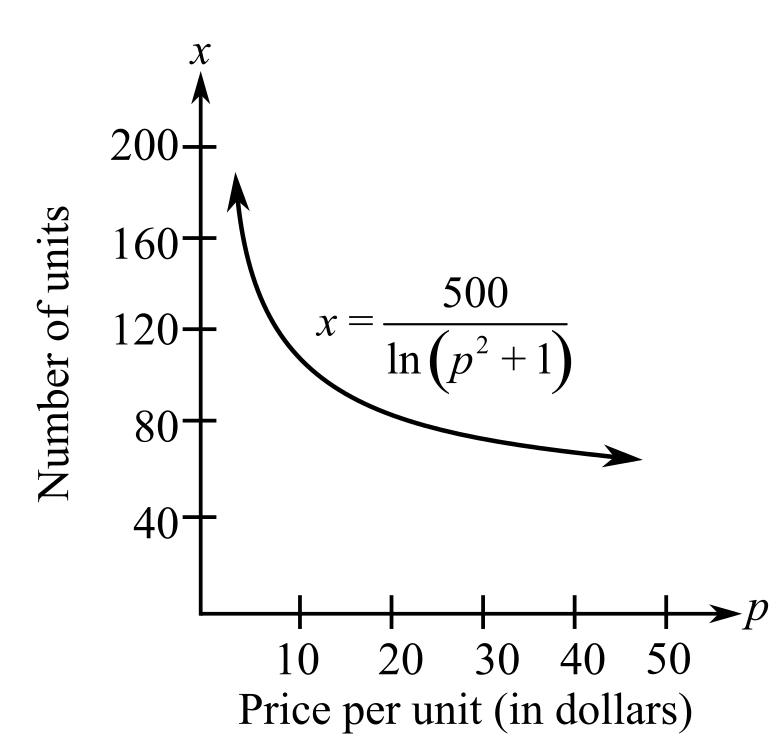Chapter 4.5, Problem 74E### Calculus: An Applied Approach (Min...

10th Edition
Ron Larson
ISBN: 9781305860919

#### Solutions

Chapter
Section### Calculus: An Applied Approach (Min...

10th Edition
Ron Larson
ISBN: 9781305860919
Textbook Problem
1 views

# Demand In Exercises 73 and 74, find dx/dp for the demand function. Interpret this rate of change for a price of $10. x = 500 ln ( p 2 + 1 )To determine To calculate: The value of dxdp for the demand function which is approximated by x=500ln(p2+1). And explain the same for the change of a price of$10 if the demand function is as follows:Explanation

Given information:

The provided function is x=500ln(p2+1) and graph is,

Formula used:

The derivative of function f(x)=un using the chain rule is:

f(x)=ddx(un)=nun1dudx

Where, u is the function of x.

Let u be a differentiable function of x then ddx[lnx]=1x,x>0 and ddx(lnu)=1ududx,u>0

As with the natural logarithm function if x and y is a positive number such that x1 and y1 then logxy=lnxlny

Calculation:

Consider the function x=500ln(p2+1),

Apply exponential properties to the function x=500ln(p2+1),

x=500ln(p2+1)x=500(ln(p2+1))1

The derivative of function x=500ln(p2+1) is calculated as follows:

Apply chain rule of derivative to the function,

dxdp=ddp500(ln(p2+1))<

### Still sussing out bartleby?

Check out a sample textbook solution.

See a sample solution

#### The Solution to Your Study Problems

Bartleby provides explanations to thousands of textbook problems written by our experts, many with advanced degrees!

Get Started